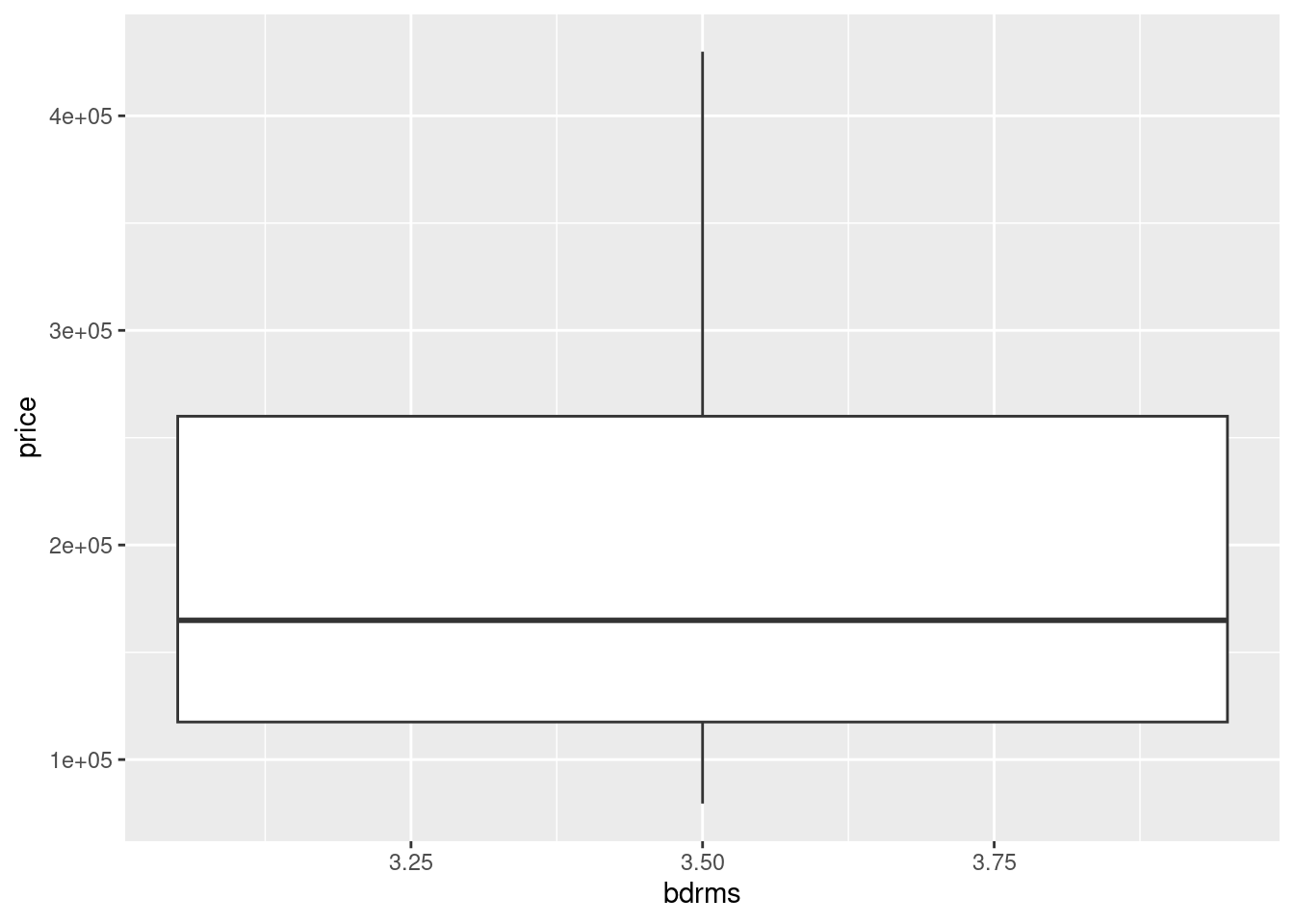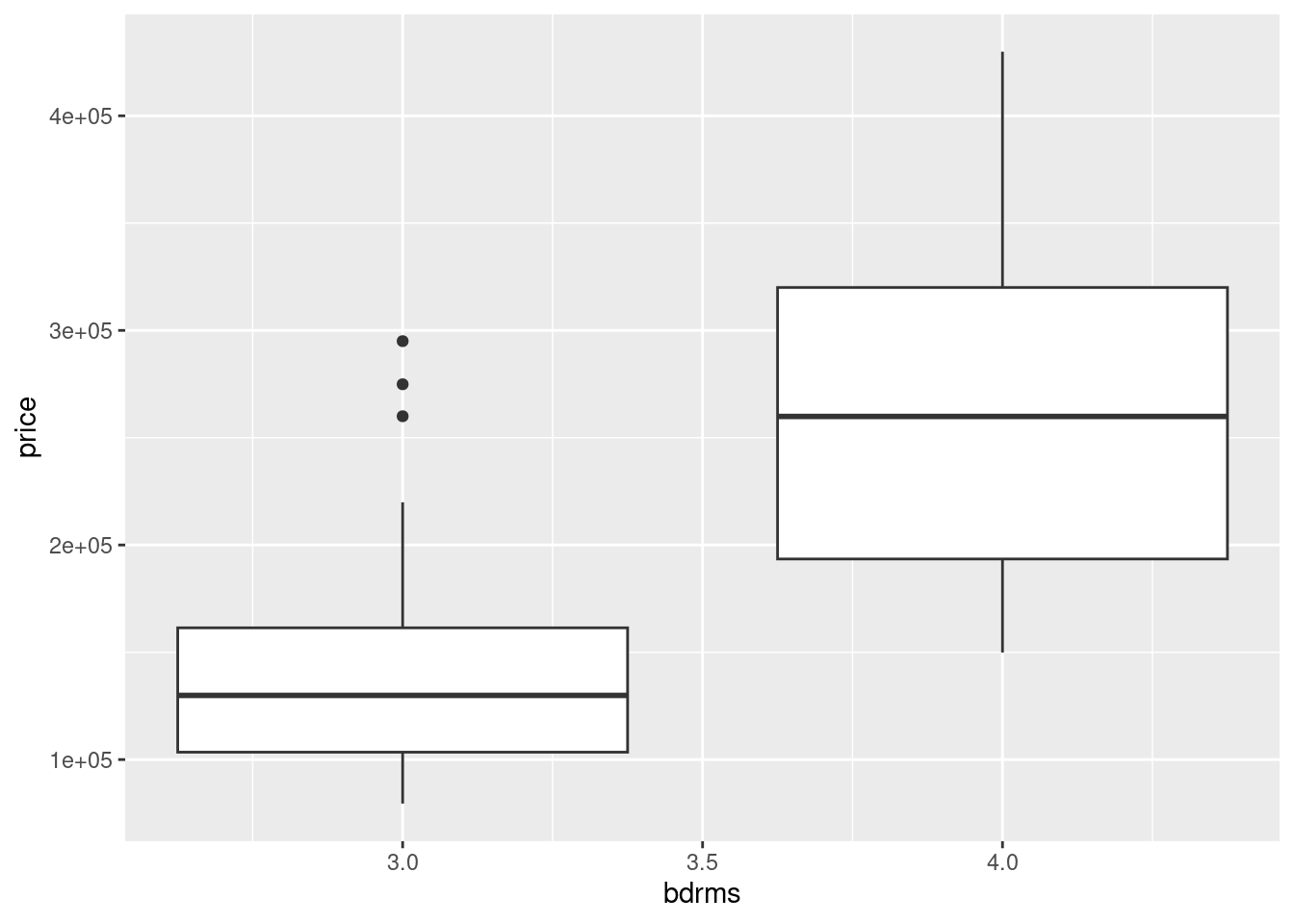# Worksheet 5

Published

September 27, 2023

Questions are below. My solutions are below all the question parts for a question; scroll down if you get stuck. There is extra discussion below that for some of the questions; you might find that interesting to read, maybe after tutorial.

For these worksheets, you will learn the most by spending a few minutes thinking about how you would answer each question before you look at my solution. There are no grades attached to these worksheets, so feel free to guess: it makes no difference at all how wrong your initial guess is!

# 1 Home prices

A realtor kept track of the asking prices of 37 homes for sale in West Lafayette, Indiana, in a particular year. The asking prices are in http://ritsokiguess.site/datafiles/homes.csv. There are two columns, the asking price (in \$) and the number of bedrooms that home has (either 3 or 4, in this dataset). The realtor was interested in whether the mean asking price for 4-bedroom homes was bigger than for 3-bedroom homes.

1. Read in and display (some of) the data.

2. Draw a suitable graph of these data.

3. Comment briefly on your plot. Does it suggest an answer to the realtor’s question? Do you have any doubts about the appropriateness of a $$t$$-test in this situation? Explain briefly.

4. Sometimes prices work better on a log scale. This is because percent changes in prices are often of more interest than absolute dollar-value changes. Re-draw your plot using logs of asking prices. (In R, log() takes natural (base $$e$$) logs, which are fine here.) Do you like the shapes of the distributions better? Hint: you have a couple of options. One is to use the log right in your plotting (or, later, testing) functions. Another is to define a new column containing the log-prices and work with that.

5. Run a suitable $$t$$-test to compare the log-prices. What do you conclude?

# My solutions

1. Read in and display (some of) the data.

Solution

The exact usual:

my_url <- "http://ritsokiguess.site/datafiles/homes.csv"
asking <- read_csv(my_url)
Rows: 37 Columns: 2
── Column specification ────────────────────────────────────────────────────────
Delimiter: ","
dbl (2): price, bdrms

ℹ Use spec() to retrieve the full column specification for this data.
ℹ Specify the column types or set show_col_types = FALSE to quiet this message.
asking

There are indeed 37 homes; the first of them have 4 bdrms, and the ones further down, if you scroll, have three. The price column does indeed look like asking prices of homes for sale.1

$$\blacksquare$$

1. Draw a suitable graph of these data.

Solution

Two groups of prices to compare, or one quantitative column and one column that appears to be categorical (it’s actually a number, but it’s playing the role of a categorical or grouping variable). So a boxplot. This requires care, though; if you do it without thinking you’ll get this:

ggplot(asking, aes(x = bdrms, y = price)) + geom_boxplot()
Warning: Continuous x aesthetic
ℹ did you forget aes(group = ...)?The message is the clue: the number of bedrooms looks quantitative and so ggplot has tried (and failed) to treat it as such.

The perhaps most direct way around this is to take the error message at face value and add bdrms as a group, thus:

ggplot(asking, aes(x = bdrms, y = price, group = bdrms)) + geom_boxplot()and that works (as you will see below, it is the same as the other methods that require a bit more thought).

You might be thinking that this is something like black magic, so I offer another idea where you have a fighting chance of understanding what is being done.

The problem is that bdrms looks like a quantitative variable (it has values 3 and 4 that are numbers), but we want it to be treated as a categorical variable. The easiest way to turn it into one is via factor, like this:

ggplot(asking, aes(x = factor(bdrms), y = price)) + geom_boxplot()